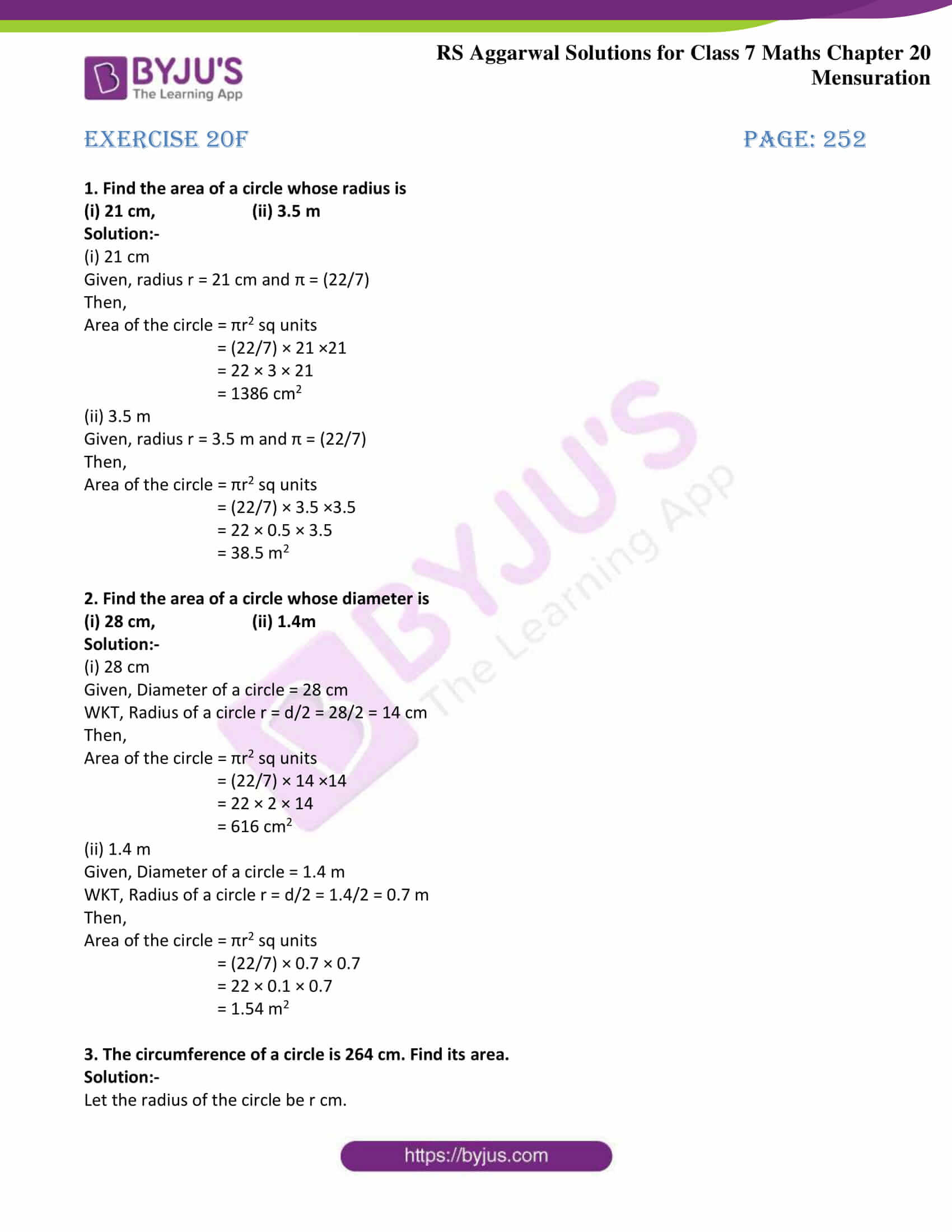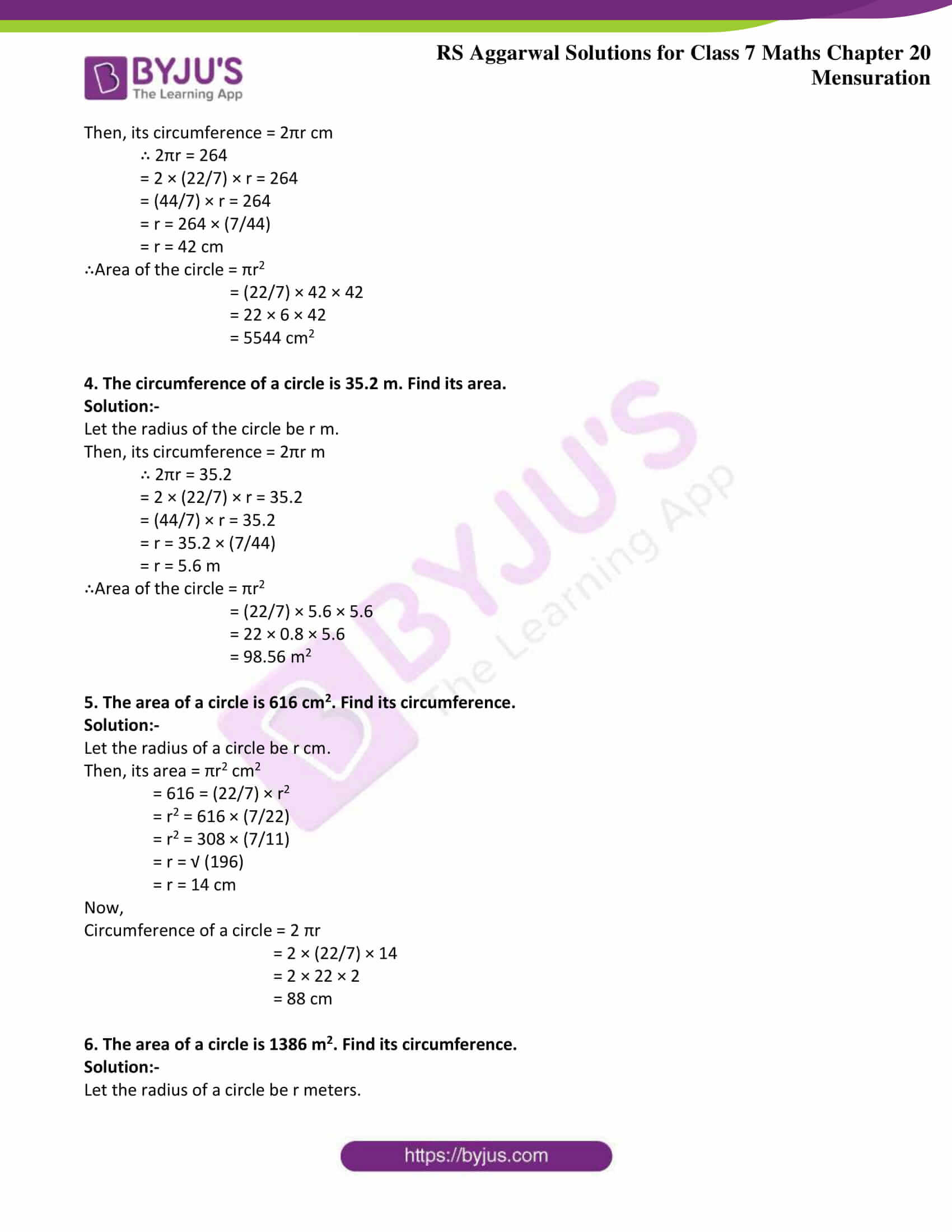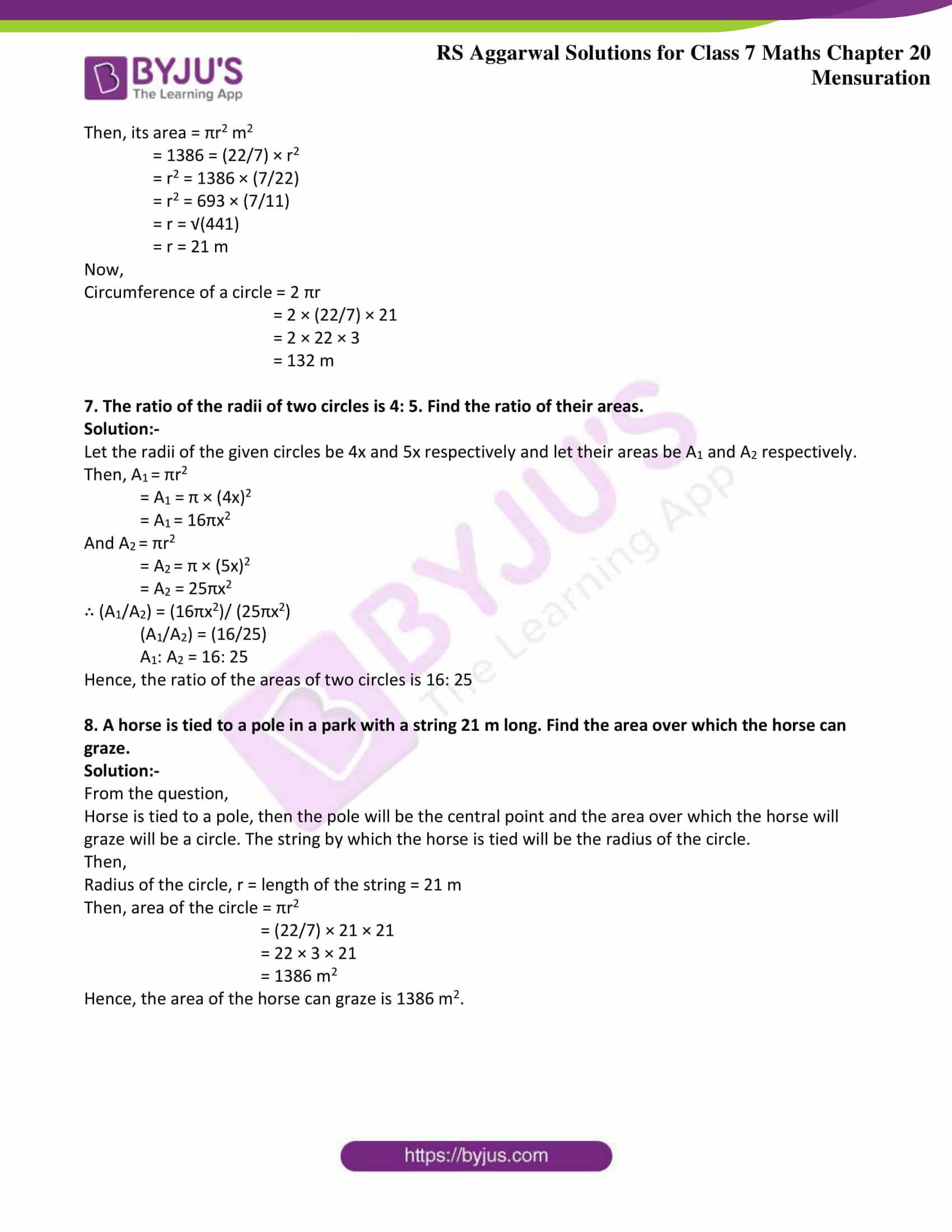# RS Aggarwal Solutions for Class 7 Maths Exercise 20F Chapter 20 Mensuration

RS Aggarwal Solutions for Class 7 Maths Exercise 20F Chapter 20 Mensuration, Students are advised to check for the solutions for each question present in this exercise and resolve the difficulties faced while solving the problems from RS Aggarwal class 7 book. This exercise of RS Aggarwal Solutions for Class 7 Chapter 20 contains questions related to the area of a circle. These RS Aggarwal Solutions for Class 7 Maths Chapter 20 Mensuration Exercise 20F, are available in downloadable PDF format so that students can download it and also learn these materials offline.

## Download the PDF of RS Aggarwal Solutions For Class 7 Maths Chapter 20 Mensuration – Exercise 20F### Access answers to Maths RS Aggarwal Solutions for Class 7 Chapter 20 – Mensuration Exercise 20F

1. Find the area of a circle whose radius is

(i) 21 cm, (ii) 3.5 m

Solution:-

(i) 21 cm

Given, radius r = 21 cm and π = (22/7)

Then,

Area of the circle = πr2 sq units

= (22/7) × 21 ×21

= 22 × 3 × 21

= 1386 cm2

(ii) 3.5 m

Given, radius r = 3.5 m and π = (22/7)

Then,

Area of the circle = πr2 sq units

= (22/7) × 3.5 ×3.5

= 22 × 0.5 × 3.5

= 38.5 m2

2. Find the area of a circle whose diameter is

(i) 28 cm, (ii) 1.4m

Solution:-

(i) 28 cm

Given, Diameter of a circle = 28 cm

WKT, Radius of a circle r = d/2 = 28/2 = 14 cm

Then,

Area of the circle = πr2 sq units

= (22/7) × 14 ×14

= 22 × 2 × 14

= 616 cm2

(ii) 1.4 m

Given, Diameter of a circle = 1.4 m

WKT, Radius of a circle r = d/2 = 1.4/2 = 0.7 m

Then,

Area of the circle = πr2 sq units

= (22/7) × 0.7 × 0.7

= 22 × 0.1 × 0.7

= 1.54 m2

3. The circumference of a circle is 264 cm. Find its area.

Solution:-

Let the radius of the circle be r cm.

Then, its circumference = 2πr cm

∴ 2πr = 264

= 2 × (22/7) × r = 264

= (44/7) × r = 264

= r = 264 × (7/44)

= r = 42 cm

∴Area of the circle = πr2

= (22/7) × 42 × 42

= 22 × 6 × 42

= 5544 cm2

4. The circumference of a circle is 35.2 m. Find its area.

Solution:-

Let the radius of the circle be r m.

Then, its circumference = 2πr m

∴ 2πr = 35.2

= 2 × (22/7) × r = 35.2

= (44/7) × r = 35.2

= r = 35.2 × (7/44)

= r = 5.6 m

∴Area of the circle = πr2

= (22/7) × 5.6 × 5.6

= 22 × 0.8 × 5.6

= 98.56 m2

5. The area of a circle is 616 cm2. Find its circumference.

Solution:-

Let the radius of a circle be r cm.

Then, its area = πr2 cm2

= 616 = (22/7) × r2

= r2 = 616 × (7/22)

= r2 = 308 × (7/11)

= r = √ (196)

= r = 14 cm

Now,

Circumference of a circle = 2 πr

= 2 × (22/7) × 14

= 2 × 22 × 2

= 88 cm

6. The area of a circle is 1386 m2. Find its circumference.

Solution:-

Let the radius of a circle be r meters.

Then, its area = πr2 m2

= 1386 = (22/7) × r2

= r2 = 1386 × (7/22)

= r2 = 693 × (7/11)

= r = √(441)

= r = 21 m

Now,

Circumference of a circle = 2 πr

= 2 × (22/7) × 21

= 2 × 22 × 3

= 132 m

7. The ratio of the radii of two circles is 4: 5. Find the ratio of their areas.

Solution:-

Let the radii of the given circles be 4x and 5x respectively and let their areas be A1 and A2 respectively.

Then, A1 = πr2

= A1 = π × (4x)2

= A1 = 16πx2

And A2 = πr2

= A2 = π × (5x)2

= A2 = 25πx2

∴ (A1/A2) = (16πx2)/ (25πx2)

(A1/A2) = (16/25)

A1: A2 = 16: 25

Hence, the ratio of the areas of two circles is 16: 25

8. A horse is tied to a pole in a park with a string 21 m long. Find the area over which the horse can graze.

Solution:-

From the question,

Horse is tied to a pole, then the pole will be the central point and the area over which the horse will graze will be a circle. The string by which the horse is tied will be the radius of the circle.

Then,

Radius of the circle, r = length of the string = 21 m

Then, area of the circle = πr2

= (22/7) × 21 × 21

= 22 × 3 × 21

= 1386 m2

Hence, the area of the horse can graze is 1386 m2.

### Access other exercises of RS Aggarwal Solutions For Class 7 Chapter 20 – Mensuration

Exercise 20A Solutions

Exercise 20B Solutions

Exercise 20C Solutions

Exercise 20D Solutions

Exercise 20E Solutions

Exercise 20G Solutions